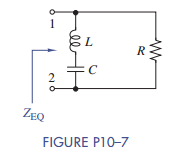### Create an Account

Home / Questions / For the circuit of Figure P10–7 a Find and express ZEQ s as a rational function and locate...

# For the circuit of Figure P10–7 a Find and express ZEQ s as a rational function and locate its poles and zeroes b If R = 1kΩ and C = 0 1 μF select a value of L to locate zeroes at

For the circuit of Figure P10–7:

(a) Find and express ZEQ(s) as a rational function and locate its poles and zeroes.

(b) If R = 1kΩ and C = 0.1 μF, select a value of L to locate zeroes at ± j10 krad/s.

(c) Where are the poles located once you have selected the inductor in part (b)?Jun 15 2020 View more View LessSubscribe To Get Solution